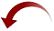# 20 Varieties of Quartiles

Published on
3,171 Points
171 Views
There is no single "correct" calculation method for quartiles. Excel features two methods, some math and statistic packages offer some more, but here is presented no less than twenty methods for various purposes using VBA and Microsoft Access.

## Quartiles

To calculate a quartile of a sample is in theory easy, and is much like calculating the median. The difficult part is the implementation; contrary to calculating the median, there exists no single specific method that stands above the rest or can be considered the "best" method among the about twenty known methods for calculating a quartile. The "best" method will be the method that fits the purpose or - in some areas - is considered a de-facto standard.

Why, how, and when to calculate quartiles using which method is outside the scope of this article. Many articles and even books covering this have been written. However, the day you face the task to calculate a quartile using some specific method, the functions here will help you.

## Methods

It is quite hard to even obtain a list of known methods for calculating a quartile, not to say proven results from these. The best source, I've located (see bottom of the article), is quite old and lists 14 methods: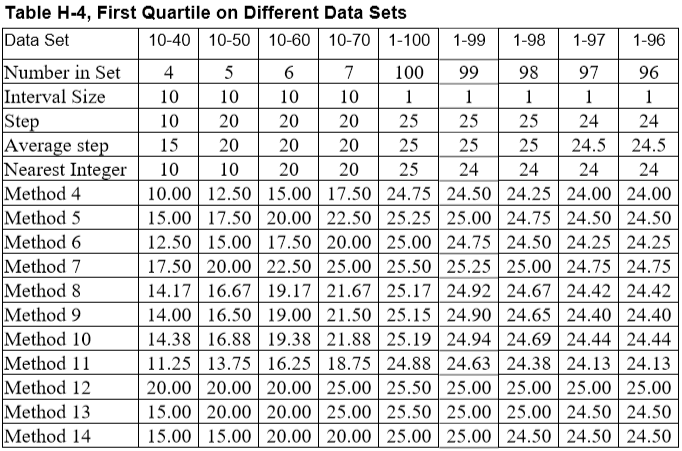The additional six methods, I have located here and there. Unfortunately, the sources have vanished.

If you are aware of any good source, please add a comment to the article.

The methods have been collected as an enum including as in-line comments their names, applications, and sources, together with their basic calculation methods for the first and the third quartile (the second is always calculated as the median):

```' Quartile calculation methods.
' Values equal those listed in the source. See function Quartile.
'
' Common names of variables used in calculation formulas.
'
' L: Q1, Lower quartile.
' H: Q3, Higher quartile.
' M: Q2, Median (not used here).
' n: Count of elements.
' p: Calculated position of quartile.
' j: Element of dataset.
' g: Decimal part of p to be used for interpolation between j and j+1.
'
Public Enum ApQuartileMethod
[_First] = 1

' Basic calculation methods.

' Step. Mendenhall and Sincich method.
'   SAS #3.
'   Round up to actual element of dataset.
'   L:  -Int(-n/4)
'   H: n-Int(-n/4)
apMendenhallSincich = 1

' Average step.
'   SAS #5, Minitab (%DESCRIBE), GLIM (percentile).    '
'   Add bias of one on basis of n/4.
'   L:   CLng((n+2)/2)/2
'   H: n-Clng((n+2)/2)/2
'   Note:
'       Replaces these original formulas that don't return the expected values.
'   L:   (Int((n+1)/4)+Int(n/4))/2+1
'   H: n-(Int((n+1)/4)+Int(n/4))/2+1
apAverage = 2

' Nearest integer to np.
'   SAS #2.
'   Round to nearest integer on basis of n/4.
'   L:   CLng(n/4)
'   H: n-CLng(n/4)
'   Note:
'       Replaces these original formulas that don't return the expected values.
'   L:   Int((n+2)/4)
'   H: n-Int((n+2)/4)
apNearestInteger = 3

' Parzen method.
'   Method 1 with interpolation.
'   SAS #1.
'   L: n/4
'   H: 3n/4
apParzen = 4

' Hazen method.
'   Values midway between method 1 steps.
'   GLIM (interpolate).
'   Wikipedia method 3.
'   Add bias of 2, don't round to actual element of dataset.
'   L: (n+2)/4
'   H: 3(n+2)/4-1
apHazen = 5

' Weibull method.
'   SAS #4. Minitab (DECRIBE), SPSS, BMDP, Excel exclusive.
'   Add bias of 1, don't round to actual element of dataset.
'   L: (n+1)/4
'   H: 3(n+1)/4
apWeibull = 6

' Freund, J. and Perles, B., Gumbell method.
'   S-PLUS, R, Excel legacy, Excel inclusive, Star Office Calc.
'   Add bias of 3, don't round to actual element of dataset.
'   L: (n+3)/4
'   H: (3n+1)/4
apFreundPerlesGumbell = 7

' Median Position.
'   Median unbiased.
'   L: (3n+5)/12
'   H: (9n+7)/12
apMedianPosition = 8

' Bernard and Bos-Levenbach.
'   L: (n/4)+0.4
'   H: (3n/4)/+0.6
'   Note:
'       Reference claims L to be (n/4)+0.31.
apBernardBosLevenbach = 9

' Blom's Plotting Position.
'   Better approximation when the distribution is normal.
'   L: (4n+7)/16
'   H: (12n+9)/16
apBlom = 10

' Moore's first method.
'   Add bias of one half step.
'   L: (n+0.5)/4
'   H: n-(n+0.5)/4
apMooreFirst = 11

' Moore's second method.
'   Add bias of one or two steps on basis of (n+1)/4.
'   L:   (Int((n+1)/4)+Int(n/4))/2+1
'   H: n-(Int((n+1)/4)+Int(n/4))/2+1
apMooreSecond = 12

' John Tukey's method.
'   Include median from odd dataset in dataset for quartile.
'   Wikipedia method 2.
'   L:   (1-Int(-n/2))/2
'   H: n-(-1-Int(-n/2))/2
apTukey = 13

' Moore and McCabe (M & M), variation of John Tukey's method.
'   TI-83.
'   Wikipedia method 1.
'   Exclude median from odd dataset in dataset for quartile.
'   L:   (Int(n/2)+1)/2
'   H: n-(Int(n/2)-1)/2
apTukeyMooreMcCabe = 14

' Additional variations between Weibull's and Hazen's methods, from
'   (i-0.000)/(n+1.00)
' to
'   (i-0.500)/(n+0.00)

' Variation of Weibull.
'   L: n(n/4-0)/(n+1)
'   H: n(3n/4-0)/(n+1)
apWeibullVariation = 15

' Variation of Blom.
'   L: n(n/4-3/8)/(n+1/4)
'   H: n(3n/4-3/8)/(n+1/4)
apBlomVariation = 16

' Variation of Tukey.
'   L: n(n/4-1/3)/(n+1/3)
'   H: n(3n/4-1/3)/(n+1/3)
apTukeyVariation = 17

' Variation of Cunnane.
'   L: n(n/4-2/5)/(n+1/5)
'   H: n(3n/4-2/5)/(n+1/5)
apCunnaneVariation = 18

' Variation of Gringorten.
'   L: n(n/4-0.44)/(n+0.12)
'   H: n(3n/4-0.44)/(n+0.12)
apGringortenVariation = 19

' Variation of Hazen.
'   L: n(n/4-1/2)/n
'   H: n(3n/4-1/2)/n
apHazenVariation = 20

[_Last] = 20
End Enum```

The actual calculation methods have been tweaked a little to fit VBA and to correct for weird results when a sample consists of very few elements.

## Functions

The main function is named Quartile and has the native domain aggregate functions, DAvg etc., in mind as it takes an Expression, a Domain, and a Criteria (filter) as arguments. Other arguments are the quartile Part to return and the Method to use:

```Expression: Name of the field or an expression to analyse.
Domain    : Name of the source/query, or an SQL select query, to analyse.
Criteria  : Optional. A filter expression for Domain.
Part      : Optional. Which median/quartile or min/max value to return.
Default is the median value.
Method    : Optional. Method for calculation of lower/higher quartile.
Default is the method by Freund, Perles, and Gumbell (Excel). ```

The function can be regarded to have four main parts:

1. Build the SQL to retrieve the ordered samples
2. Calculate either the minimum or maximum value, the first or third quartile, or the median
3. Prepare for interpolation
4. Calculate the final output

```Public Function Quartile( _
ByVal Expression As String, _
ByVal Domain As String, _
Optional ByVal Criteria As String, _
Optional ByVal Part As ApQuartilePart = ApQuartilePart.apMedian, _
Optional ByVal Method As ApQuartileMethod = ApQuartileMethod.apFreundPerlesGumbell) _
As Double

' SQL.
Const SqlMask           As String = "Select {0} From {1} {2}"
Const SqlLead           As String = "Select "
Const SubMask           As String = "({0}) As T"
Const FilterMask        As String = "Where {0} "
Const OrderByMask       As String = "Order By {0} Asc"

Dim Records     As DAO.Recordset

Dim Sql         As String
Dim SqlSub      As String
Dim Filter      As String
Dim Count       As Long     ' n.
Dim Position    As Double   ' p.
Dim Element     As Long     ' j.
Dim Interpolate As Double   ' g.
Dim ValueOne    As Double
Dim ValueTwo    As Double
Dim Value       As Double

' Return default quartile part if choice of part is
' outside the range of ApQuartilePart.
If Not IsQuartilePart(Part) Then
Part = ApQuartilePart.apMedian
End If

' Use a default calculation method if choice of method is
' outside the range of ApQuartileMethod.
If Not IsQuartileMethod(Method) Then
Method = ApQuartileMethod.apFreundPerlesGumbell
End If

If Domain <> "" And Expression <> "" Then
' Build SQL to lookup values.
If InStr(1, LTrim(Domain), SqlLead, vbTextCompare) = 1 Then
' Domain is an SQL expression.
Else
' Domain is a table or query name.
SqlSub = Domain
End If
If Trim(Criteria) <> "" Then
' Build Where clause.
End If
' Build final SQL.
Sql = Replace(Replace(Replace(SqlMask, "{0}", Expression), "{1}", SqlSub), "{2}", Filter) & _
Set Records = CurrentDb.OpenRecordset(Sql, dbOpenSnapshot)

With Records
If Not .EOF = True Then
If Part = ApQuartilePart.apMinimum Then
' No need to count records.
Count = 1
Else
' Count records.
.MoveLast
Count = .RecordCount
End If
Select Case Part
Case ApQuartilePart.apMinimum
' Current record is first record.
' Read value of this record.
Case ApQuartilePart.apMaximum
' Current record is last record.
' Read value of this record.
Case ApQuartilePart.apMedian
' Locate position of median.
Position = (Count + 1) / 2
Case ApQuartilePart.apLower
Select Case Method
Case ApQuartileMethod.apMendenhallSincich
Position = -Int(-Count / 4)
Case ApQuartileMethod.apAverage
Position = CLng((Count + 2) / 2) / 2
Case ApQuartileMethod.apNearestInteger
Position = CLng(Count / 4)
Case ApQuartileMethod.apParzen
Position = Count / 4
Case ApQuartileMethod.apHazen
Position = (Count + 2) / 4
Case ApQuartileMethod.apWeibull
Position = (Count + 1) / 4
Case ApQuartileMethod.apFreundPerlesGumbell
Position = (Count + 3) / 4
Case ApQuartileMethod.apMedianPosition
Position = (3 * Count + 5) / 12
Case ApQuartileMethod.apBernardBosLevenbach
Position = (Count / 4) + 0.4
Case ApQuartileMethod.apBlom
Position = (4 * Count + 7) / 16
Case ApQuartileMethod.apMooreFirst
Position = (Count + 0.5) / 4
Case ApQuartileMethod.apMooreSecond
Position = (Int((Count + 1) / 4) + Int(Count / 4)) / 2 + 1
Case ApQuartileMethod.apTukey
Position = (1 - Int(-Count / 2)) / 2
Case ApQuartileMethod.apTukeyMooreMcCabe
Position = (Int(Count / 2) + 1) / 2
Case ApQuartileMethod.apWeibullVariation
Position = Count * (Count / 4) / (Count + 1)
Case ApQuartileMethod.apBlomVariation
Position = Count * (Count / 4 - 3 / 8) / (Count + 1 / 4)
Case ApQuartileMethod.apTukeyVariation
Position = Count * (Count / 4 - 1 / 3) / (Count + 1 / 3)
Case ApQuartileMethod.apCunnaneVariation
Position = Count * (Count / 4 - 2 / 5) / (Count + 1 / 5)
Case ApQuartileMethod.apGringortenVariation
Position = Count * (Count / 4 - 0.44) / (Count + 0.12)
Case ApQuartileMethod.apHazenVariation
Position = Count * (Count / 4 - 1 / 2) / Count
End Select
Case ApQuartilePart.apUpper
' Default position for very low counts for several methods
Position = Count
Select Case Method
Case ApQuartileMethod.apMendenhallSincich
If Count > 2 Then
Position = Count - (-Int(-Count / 4))
End If
Case ApQuartileMethod.apAverage
If Count > 2 Then
Position = Count - CLng((Count + 2) / 2) / 2
End If
Case ApQuartileMethod.apNearestInteger
Position = Count - CLng(Count / 4)
Case ApQuartileMethod.apParzen
Position = 3 * Count / 4
Case ApQuartileMethod.apHazen
If Count > 1 Then
Position = 3 * (Count + 2) / 4 - 1
End If
Case ApQuartileMethod.apWeibull
If Count > 2 Then
Position = 3 * (Count + 1) / 4
End If
Case ApQuartileMethod.apFreundPerlesGumbell
Position = (3 * Count + 1) / 4
Case ApQuartileMethod.apMedianPosition
If Count > 2 Then
Position = (9 * Count + 7) / 12
End If
Case ApQuartileMethod.apBernardBosLevenbach
If Count > 2 Then
Position = (3 * Count / 4) + 0.6
End If
Case ApQuartileMethod.apBlom
If Count > 2 Then
Position = (12 * Count + 9) / 16
End If
Case ApQuartileMethod.apMooreFirst
Position = Count - (Count + 0.5) / 4
Case ApQuartileMethod.apMooreSecond
' Basic calculation method. Will fail for 2 or 3 elements.
'   Position = Count - (Int((Count + 1) / 4) + Int(Count / 4)) / 2 + 1
' Calculation method adjusted to accept 2 or 3 elements.
Position = Count - (Int((Count + Int((Count * 2) / (Count + 4))) / 4) + Int(Count / 4)) / 2 + 1
Case ApQuartileMethod.apTukey
Position = Count - (-1 - Int(-Count / 2)) / 2
Case ApQuartileMethod.apTukeyMooreMcCabe
If Count > 1 Then
Position = Count - (Int(Count / 2) - 1) / 2
End If
Case ApQuartileMethod.apWeibullVariation
Position = Count * (3 * Count / 4) / (Count + 1)
Case ApQuartileMethod.apBlomVariation
Position = Count * (3 * Count / 4 - 3 / 8) / (Count + 1 / 4)
Case ApQuartileMethod.apTukeyVariation
Position = Count * (3 * Count / 4 - 1 / 3) / (Count + 1 / 3)
Case ApQuartileMethod.apCunnaneVariation
Position = Count * (3 * Count / 4 - 2 / 5) / (Count + 1 / 5)
Case ApQuartileMethod.apGringortenVariation
Position = Count * (3 * Count / 4 - 0.44) / (Count + 0.12)
Case ApQuartileMethod.apHazenVariation
Position = Count * (3 * Count / 4 - 1 / 2) / Count
End Select
End Select
Select Case Part
Case ApQuartilePart.apMinimum, ApQuartilePart.apMaximum
Case Else
.MoveFirst
' Find position of first observation to retrieve.
' If Element is 0, then upper position is first record.
' If Element is not 0 and position is not an integer, then
' read the next observation too.
Element = Fix(Position)
Interpolate = Position - Element
If Count = 1 Then
' Nowhere else to move.
If Interpolate < 0 Then
' Prevent values to be created by extrapolation beyond zero from observation one
' for these methods:
'   ApQuartileMethod.apBlomVariation
'   ApQuartileMethod.apTukeyVariation
'   ApQuartileMethod.apCunnaneVariation
'   ApQuartileMethod.apGringortenVariation
'   ApQuartileMethod.apHazenVariation
'
' Comment this line out, if reading by extrapolation *is* requested.
Interpolate = 0
End If
ElseIf Element > 1 Then
' Move to the record to read.
.Move Element - 1
' Special case for apMooreSecond and upper quartile for 2 and 3 elements.
If .EOF Then
.MoveLast
End If
End If
End Select
' Retrieve value from first observation.
ValueOne = .Fields(0).Value

Select Case Part
Case ApQuartilePart.apMinimum, ApQuartilePart.apMaximum
Value = ValueOne
Case Else
If Interpolate = 0 Then
' Only one observation to read.
If Element = 0 Then
' Return 0.
Else
Value = ValueOne
End If
Else
If Element = 0 Or Element = Count Then
' No first/last observation to retrieve.
ValueTwo = ValueOne
If ValueOne > 0 Then
' Use 0 as other observation.
ValueOne = 0
Else
ValueOne = 2 * ValueOne
End If
Else
' Move to next observation.
.MoveNext
' Retrieve value from second observation.
ValueTwo = .Fields(0).Value
End If
' For positive values interpolate between 0 and ValueOne.
' For negative values interpolate between 2 * ValueOne and ValueOne.
' Calculate quartile using linear interpolation.
Value = ValueOne + Interpolate * CDec(ValueTwo - ValueOne)
End If
End Select
End If
.Close
End With
End If

Quartile = Value

End Function```

Two important features are, that the Domain argument can be an SQL select query, and the samples in the passed records do not have to be sorted. The function will itself take care of sorting the samples.

Thus, typical usages can be as listed here where the resulting SQL has been included for better understanding of the parsing of the Domain argument done by the function:

```' Example calls and the internally generated SQL:
'
'   With fieldname as expression, table (or query) as domain, no filter, and default sorting:
'       Q1 = Quartile("Data", "Observation", , apFirst, apFreundPerlesGumbell)
'       Select Data From Observation Order By Data Asc
'
'   With two fieldnames as expression, table (or query) as domain, no filter, and sorting on two fields:
'       Q1 = Quartile("Data, Step", "Observation", , apFirst, apFreundPerlesGumbell)
'       Select Data, Step From Observation Order By Data, Step Asc
'
'   With fieldname as expression, SQL as domain, no filter, and default sorting:
'       Q1 = Quartile("Data", "Select Data From Observation", , apFirst, apFreundPerlesGumbell)
'       Select Data From (Select Data From Observation) As T Order By Data Asc
'
'   With fieldname as expression, SQL as domain, simple filter, and sorting on one field:
'       Q1 = Quartile("Data", "Select Data, Step From Observation", "Step = 10", apFirst, apFreundPerlesGumbell)
'       Select Data From (Select Data, Step From Observation) As T Where Step = 10 Order By Data Asc
'
'   With calculated expression, SQL as domain, extended filter, and sorting on one field:
'       Q1 = Quartile("Data * 10", "Select Data, Step From Observation", "Step = 10 And Data <= 40", apFirst, apFreundPerlesGumbell)
'       Select Data * 10 From (Select Data, Step From Observation) As T Where Step = 10 And Data <= 40 Order By Data * 10 Asc
'
'   With filtered SQL domain, additional filter, and sorting on one field:
'       Q1 = Quartile("Data", "Select Data, Step From Observation Where Step = 10", "Data <= 40", apFirst, apFreundPerlesGumbell)
'       Select Data From (Select Data, Step From Observation Where Step = 10) As T Where Data <= 40 Order By Data Asc
'
'   With filtered SQL domain, additional filter, and sorting on two fields:
'       Q1 = Quartile("Step, Data", "Select Data, Step From Observation Where Step = 10", "Data <= 40", apFirst, apFreundPerlesGumbell)
'       Select Step, Data From (Select Data, Step From Observation Where Step = 10) As T Where Data <= 40 Order By Step, Data Asc```

Note please, that the function is heavily in-line documented as the code otherwise would be uncomprehensive.

## Domain functions

To ease the use, indeed in queries, two domain functions supplement the main function:

DMedian

DQuartile

These mimic the native Dxxx domain aggregate functions and take only the arguments needed, using default values - for DMedian, for the part to return and, for DQuartile, for the calculation method to use; that method has been chosen to be the original method used by Excel (formulas QUARTILE and QUARTILE.INCL):

```' Returns the median of a field of a table/query.
'
' Parameters:
'   Expression: Name of the field or an expression to analyse.
'   Domain    : Name of the source/query, or an SQL select query, to analyse.
'   Criteria  : Optional. A filter expression for Domain.
'
' Reference and examples: See function Quartile.
'
' Data must be in ascending order by Field.
'
' 2019-08-15. Gustav Brock, Cactus Data ApS, CPH.
'
Public Function DMedian( _
ByVal Expression As String, _
ByVal Domain As String, _
Optional ByVal Criteria As String) _
As Double

Dim Value       As Double

Value = Quartile(Expression, Domain, Criteria)

DMedian = Value

End Function```
```' Returns the upper or lower quartile or the median or the
' minimum or maximum value of a field of a table/query
' using the method by Freund, Perles, and Gumbell (Excel).
'
' Parameters:
'   Expression: Name of the field or an expression to analyse.
'   Domain    : Name of the source/query, or an SQL select query, to analyse.
'   Criteria  : Optional. A filter expression for Domain.
'   Part      : Optional. Which median/quartile or min/max value to return.
'               Default is the median value.
'
' Reference and examples: See function Quartile.
'
' 2019-08-15. Gustav Brock, Cactus Data ApS, CPH.
'
Public Function DQuartile( _
ByVal Expression As String, _
ByVal Domain As String, _
Optional ByVal Criteria As String, _
Optional ByVal Part As ApQuartilePart = ApQuartilePart.apMedian) _
As Double

Dim Value       As Double

Value = Quartile(Expression, Domain, Criteria, Part)

DQuartile = Value

End Function```

## Results

An example workbook with generated results from the Excel formulas is attached for reference.

It displays like this: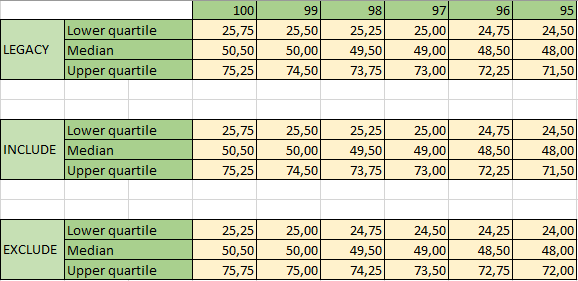The output from the function ListExcelQuartile, found in the attached Access example file, lists identical values.

The two methods are our methods 7 and 6, or the enum elements apFreundPerlesGumbell and apWeibull:

```               100           99            98            97            96            95
INCLUDE (LEGACY)
7            25,75         25,50         25,25         25,00         24,75         24,50
7            50,50         50,00         49,50         49,00         48,50         48,00
7            75,25         74,50         73,75         73,00         72,25         71,50

EXCLUDE
6            25,25         25,00         24,75         24,50         24,25         24,00
6            50,50         50,00         49,50         49,00         48,50         48,00
6            75,75         75,00         74,25         73,50         72,75         72,00 ```

Likewise, the function ListFirstQuartile returns an output similar to the results from the main source (table H-4 at top):

```               40            50            60            70
1            10,00         20,00         20,00         20,00
2            15,00         20,00         20,00         20,00
3            10,00         10,00         20,00         20,00
4            10,00         12,50         15,00         17,50
5            15,00         17,50         20,00         22,50
6            12,50         15,00         17,50         20,00
7            17,50         20,00         22,50         25,00
8            14,17         16,67         19,17         21,67
9            14,00         16,50         19,00         21,50
10           14,38         16,88         19,38         21,88
11           11,25         13,75         16,25         18,75
12           20,00         20,00         20,00         25,00
13           15,00         20,00         20,00         25,00
14           15,00         15,00         20,00         20,00
15           8,00          10,42         12,86         15,31
16           5,88          8,33          10,80         13,28
17           6,15          8,59          11,05         13,52
18           5,71          8,17          10,65         13,13
19           5,44          7,91          10,39         12,88
20           5,00          7,50          10,00         12,50

100           99            98            97            96            95
1            25,00         25,00         25,00         25,00         24,00         24,00
2            25,50         25,00         25,00         25,00         24,50         24,00
3            25,00         25,00         24,00         24,00         24,00         24,00
4            25,00         24,75         24,50         24,25         24,00         23,75
5            25,50         25,25         25,00         24,75         24,50         24,25
6            25,25         25,00         24,75         24,50         24,25         24,00
7            25,75         25,50         25,25         25,00         24,75         24,50
8            25,42         25,17         24,92         24,67         24,42         24,17
9            25,40         25,15         24,90         24,65         24,40         24,15
10           25,44         25,19         24,94         24,69         24,44         24,19
11           25,13         24,88         24,63         24,38         24,13         23,88
12           26,00         25,50         25,00         25,00         25,00         24,50
13           25,50         25,50         25,00         25,00         24,50         24,50
14           25,50         25,00         25,00         24,50         24,50         24,00
15           24,75         24,50         24,25         24,00         23,75         23,50
16           24,56         24,31         24,06         23,81         23,56         23,31
17           24,58         24,33         24,08         23,83         23,58         23,33
18           24,55         24,30         24,05         23,80         23,55         23,30
19           24,53         24,28         24,03         23,78         23,53         23,28
20           24,50         24,25         24,00         23,75         23,50         23,25         ```

Note please, that column 100-96 here contain the correct values, while in Table H-4 they hold the values for samples 99-95.

The two small examples found on Wikipedia display the results using three different methods which equal our methods 14, 13, and 5 respectively, or the enum elements apTukeyMooreMcCabe, apTukey, and apHazen:

#### Example 2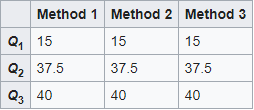These can be reproduced by the function ListWikipediaSamples:

```              Method 1      Method 2      Method 3

Q1             15            25,5          20,25
Q2             40            40            40
Q3             43            42,5          42,75

Q1             15            15            15
Q2             37,5          37,5          37,5
Q3             40            40            40 ```

Also, a query, FirstQuartileAllMethods, is included which will list the results for all sets of samples between 1 and 100 for all 20 methods for the lower quartile. Here's a snip: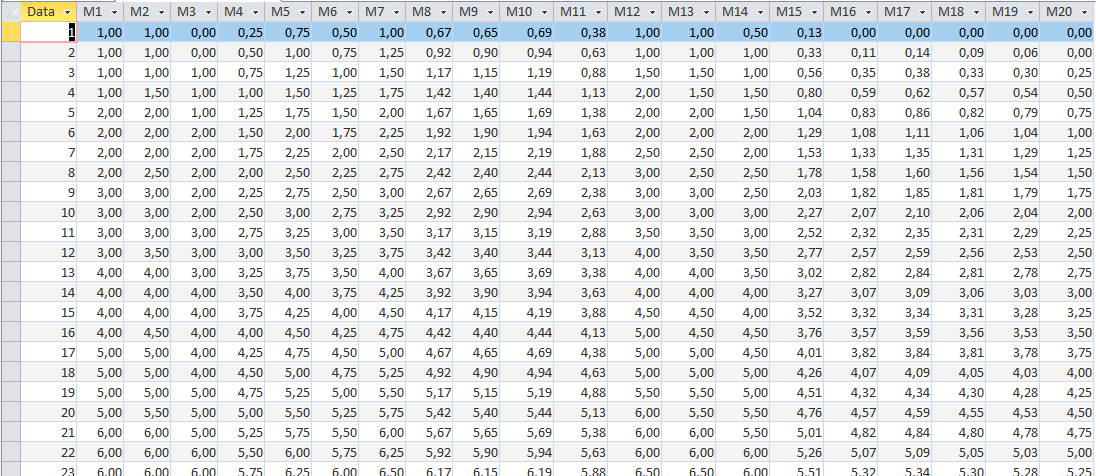Finally, a form is included which lets you select any method and then have the results for all three quartiles for every sample between 1 and 100 listed: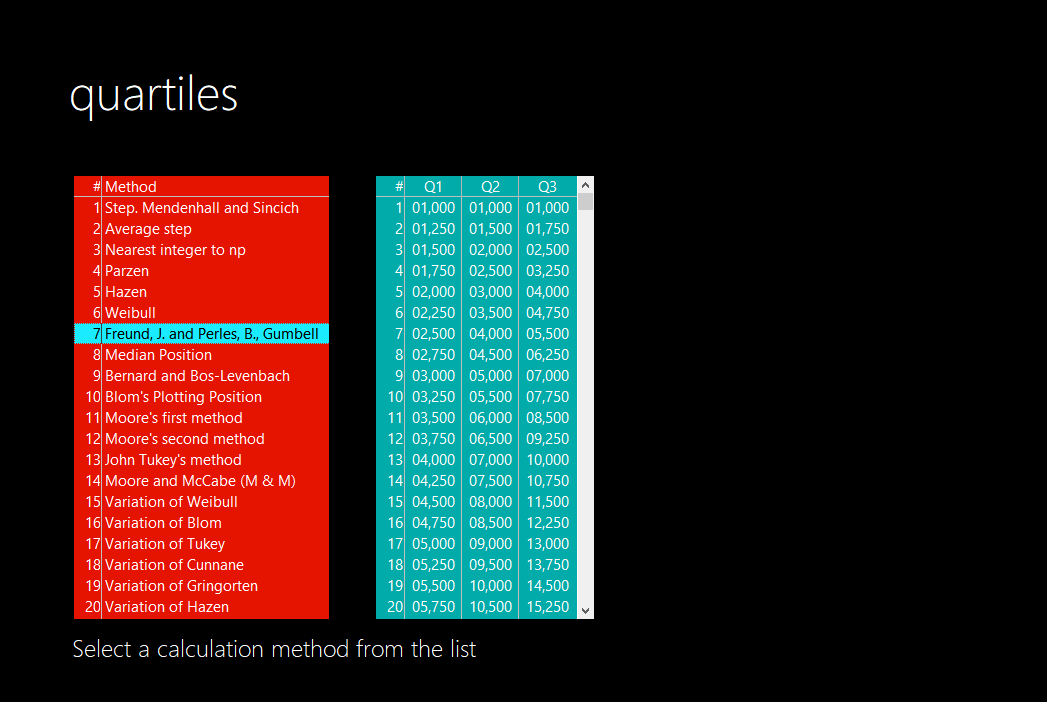## Implementation

To be able to calculate quartiles, import the module QuartileCode in your application. That's all.

The other module, QuartileDemo, is only needed for testing and for the demo form (also named QuartileDemo) to display.

Bonus tip: Study the form's code to see how to right-align numbers in a Listbox column.

## Conclusion

From the sparse sources to be located, a function has been created that for just about any practical purpose will allow for the quartiles of a sample of records to be calculated by twenty different methods.

In addition, simplified functions intended to supplement the native domain aggregate functions have been presented. Also, a collection of functions and a query for testing and demonstration have been included.

## Sources

Original source (now off-line) by David A. Heiser: http://www.daheiser.info/excel/notes/NOTE%20N.pdf

Archived source at The Internet Archive: NOTE 20
Notes:

1. Table H-4, p. 4, has correct data for the dataset for 1-96 while the datasets for 1-100 to 1-97 actually are the datasets for 1-99 to 1-96 shifted one column left. Thus, the dataset for 1-100 is missing, and that for 1-96 is listed twice.
2. Method 3b is not implemented as no one seems to use it. Neither is no example data given. Thus method 3a has here been labeled method

Further notes on quartiles and methods can be found here:

Wikipedia

Math Forum

HaiWeb

murdoch.edu.au (archived)

Should you be aware of any good source that can supplement or improve this article, please do not hesitate posting a link as comment.

The full and current code is available for download at GitHub: VBA.Quartiles

Also, code and a demo application is here: Quartiles 1.0.1.zip

An Excel workbook with the presented example: Quartiles.xlsx